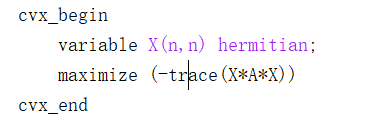# How do I solve a problem with the Trace of quadratic formIn which A is a Hermitian matrix.
The error occurs as: Disciplined convex programming error:
Only scalar quadratic forms can be specified in CVX
I was wondering if it is possible to solve it via CVX ?

Apply the same transformation as in this answer you “liked” Help me for this objective function .

`maximize(-square_pos(norm(sqrtm(A)*X,'fro')))`

The argmax is preserved if instead you use `maximize(-norm(sqrtm(A)*X,'fro'))` which avoids unnecessary squarng, and might be better behaved numerically.

Thank you very much，I am a newbie in this community, and I found your answer after I asked the question. It realy helps a lot in my work.
Best wishes .